### Home > CALC > Chapter 1 > Lesson 1.3.2 > Problem1-114

1-114.
1. State the domain for each of the following functions. Homework Help ✎

1.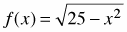2.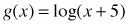3.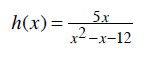4.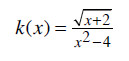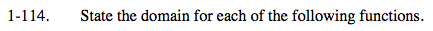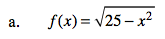You should recognize that f(x) is the equation of a semi-circle with radius 5 and center at the origin.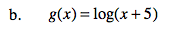The parent function, y = logx, has an open domain of 0 < x > ∞.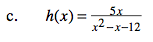Factor the denominator. Remember, that the domain cannot support zeros in the denominator.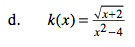The numerator and denominator will be restrictedin different ways. Combine both restrictions.

Domain: x > −2, x ≠ 2